# hasChangedFrom

## 语法

``tf = hasChangedFrom(data,value)``

## 描述

`如果 `data` 的值等于在上一时间步开始时的 `value` 并且不同于在当前时间步开始时的值，则 `tf = hasChangedFrom(data,value)` 返回 `1` (`true`)。否则，运算符返回 `0` (`false`)。`

## 示例

`[hasChangedFrom(M,matrixValue)]`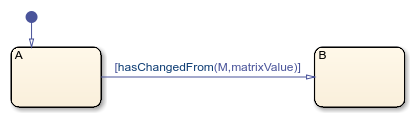`[hasChangedFrom(M(1,3),7)]`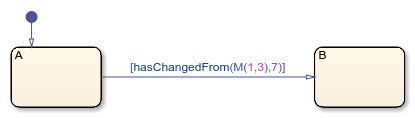`[hasChangedFrom(M,7)]`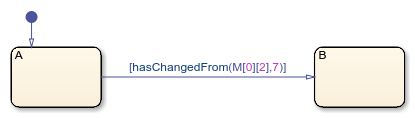`[hasChangedFrom(struct,structValue)]`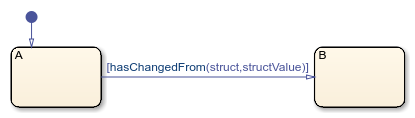`[hasChangedFrom(struct.field,5)]`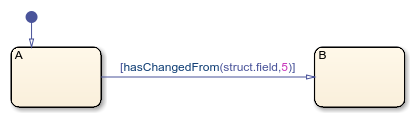## 输入参数

Stateflow® 数据，指定为：

• 标量

• 矩阵或矩阵的元素

• 结构体或结构体中的字段

• 结构体字段或矩阵元素的有效组合

MATLAB 中的独立图不支持对矩阵的元素或结构体中的字段进行更改检测。

• 如果 `data` 是标量，则 `value` 必须解析为标量。

• 如果 `data` 是矩阵，则 `value` 必须解析为与 `data` 具有相同维度的矩阵。

在使用 C 语言作为动作语言的图中，`value` 也可以解析为标量值。图通过标量扩展将 `data` 与一个矩阵进行比较，该矩阵的每个元素都等于 `value` 指定的值。请参阅为矩阵的所有元素赋值

• 如果 `data` 是结构体，则 `value` 必须解析为一个字段设定与 `data` 完全匹配的结构体。

## 提示

• 如果多个输入事件在同一时间步内发生，`hasChangedFrom` 运算符可以检测输入事件之间数据值的更改。

• 如果图写入数据对象，但不更改数据值，则 `hasChangedFrom` 运算符返回 `false`

• Stateflow 图的类型确定更改检测运算符支持的数据的作用域：

• MATLAB 中的独立 Stateflow 图：仅限“`局部`

• 在 Simulink® 模型中，使用 MATLAB 作为动作语言的图：仅限“`输入`

• 在 Simulink 模型中，使用 C 语言作为动作语言的图：“`输入`”、“`输出`”、“`局部`”或“`数据存储内存`

• 在 MATLAB 中的独立图中，更改检测运算符可以检测在对 `step` 函数的调用中指定的数据更改，因为这些更改发生在当前时间步开始之前。例如，如果 `x` 等于零，则当您使用以下命令执行图 `ch` 时，表达式 `hasChangedFrom(x,0)` 返回 `true`

`step(ch,x=1);`
相比之下，更改检测运算符无法检测由同一时间步中的状态或转移动作中的赋值引起的数据更改。此时，运算符在下一个时间步开始时检测值的更改。

• 在 Simulink 模型的图中，如果启用图选项每次图唤醒时初始化输出，则使用输出作为 `hasChanged` 运算符的参数将始终返回 `false`。有关详细信息，请参阅每次图唤醒时初始化输出

• 在使用 `hasChangedFrom` 的图中启用行优先数组布局时，代码生成会产生错误。在使用 `hasChangedFrom` 的图中生成代码之前，请启用列优先数组布局。请参阅Select Array Layout for Matrices in Generated Code